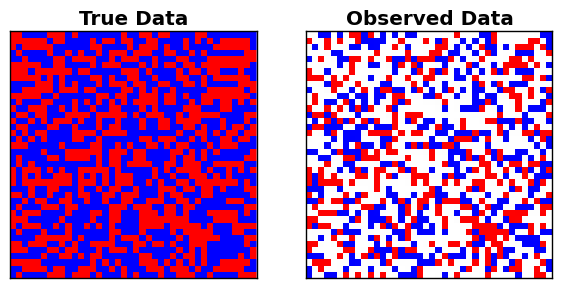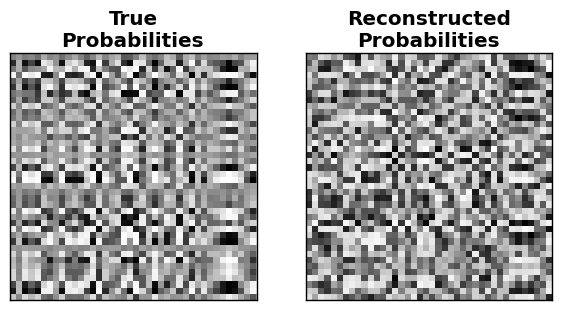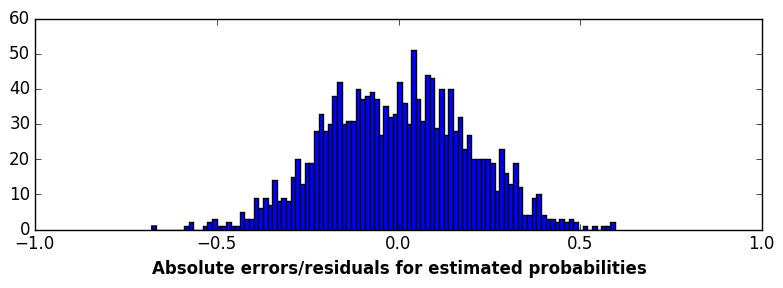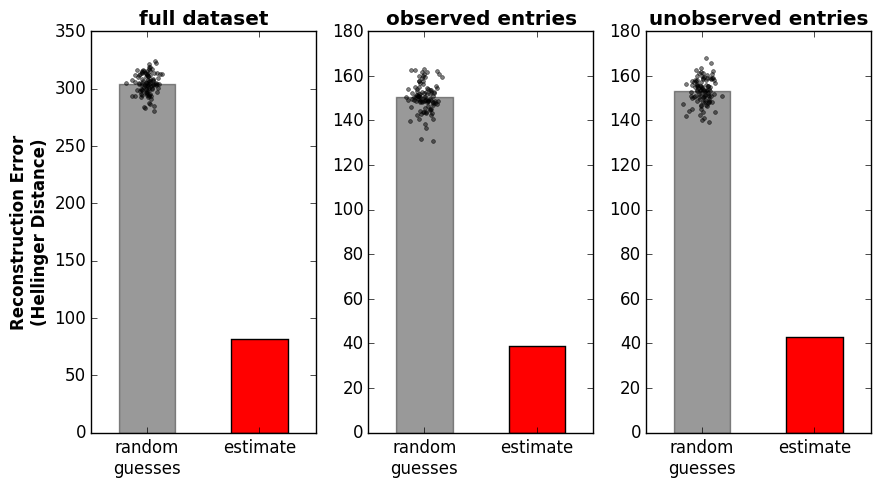# Binary Matrix Completion in Julia¶

### Author: Alex Williams¶

License:### Relevent papers¶

Mark A. Davenport, Yaniv Plan, Ewout van den Berg, Mary Wootters. 1-Bit Matrix Completion Information and Inference 3(3), 189-223. (2014)

### Relevent Code¶

Github Repo: Convex.jl

Paper: Madeleine Udell, Karanveer Mohan, David Zeng, Jenny Hong, Steven Diamond, Stephen Boyd. Convex Optimization in Julia. SC14 Workshop on High Performance Technical Computing in Dynamic Languages

### Math Notes¶

Let $Y$ be a binary matrix with missing entries. We will fit a model $Y \approx f(X)$, where $X$ is a matrix of real numbers and $f$ is a function that maps real numbers into probabilities, $\mathbf{R} \rightarrow [0,1]$, and is applied element-wise to $X$. Let $\Omega$ be the set of matrix indices $(i,j)$ that are observed in $Y$.

We will consider the case where $f(\cdot)$ is the logistic function:

$$f(x) = \frac{1}{1+\exp(-x)}$$

The log-likelihood function for a given estimate of $X$ is:

$$\mathcal{L}(X|Y) = \sum_\Omega \left [ \mathcal{I}_{Y_{ij} = 1} \log\left(f(X_{ij})\right) + \mathcal{I}_{Y_{ij} = -1} \log\left(1 -f(X_{ij})\right) \right ]$$

Where $\mathcal{I}$ is the binary event indicator function. Because $f(-x) = 1-f(x)$ for the logistic function, the likelihood simplifies to:

$$\mathcal{L}(X|Y) = \sum_\Omega - \log \left [ 1 + \exp(-Y_{ij} X_{ij} )\right ]$$

Our goal is to maximize find an $X$ that maximizes the likelihood. However, the problem is under-determined due to the missing entries in $Y$. Thus, we search for an $X$ that is low-rank, which we achieve by imposing a nuclear norm constraint.

\begin{aligned} & \underset{X}{\text{minimize}} & & \sum_{(i,j) \in \Omega} \log(1+\exp(-Y_{ij} (X_{ij})) \\ & \text{subject to} & & ||X||_* \leq \gamma \end{aligned}
In :
using PyPlot
using Convex
using SCS

# passing in verbose=0 to hide output from SCS
solver = SCSSolver(verbose=0)
set_default_solver(solver);

# logistic function
lgc(x) = 1 ./ (1+exp(-x));

INFO: Loading help data...

In :
# Construct a random m-by-n binary matrix matrix
m,n,k = 40,40,2
M = randn(m,k)*randn(k,n)       # Underlying low-rank matrix
fM = lgc(M)                     # Probability matrix, maps M onto interval [0,1]
Y = map(x->x>rand()? 1 : -1,fM) # True data, binary matrix

# Suppose that we only observe a fraction of entries in Y
n_obs = 800
Yobs = fill(NaN,(m,n))
obs = randperm(m*n)[1:n_obs]
Yobs[obs] = Y[obs]

# Plot the ground-truth, full dataset and our partial observations
figure(figsize=(7,14))
subplot(1,2,1)
imshow(Y,cmap=ColorMap("bwr"),interpolation="None")
xticks([]),yticks([]),title("True Data",fontweight="bold")

subplot(1,2,2)
imshow(Yobs,cmap=ColorMap("bwr"),interpolation="None")
xticks([]),yticks([]),title("Observed Data",fontweight="bold")
show()In :
# Reconstruct the full matrix, via nuclear norm minimization
Mest = Variable(m,n) # estimate of matrix M
p = minimize(logistic_loss(-Y[obs].*(Mest[obs])))
p.constraints += nuclear_norm(Mest) <= trace(real(sqrtm(M'*M))) # impose low-rank on estimate of M
solve!(p)


## Each observation, $Y_{ij}$, is a stochastic event. We are interested in the probability associated with these events.¶

### Put differently, $Y_{ij} \sim \text{Bernoulli}(P_{ij})$. How good is our estimate of $P_{ij}$?¶

In :
fMest = lgc(Mest.value)

# Plot the ground-truth vs our reconstruction
figure(figsize=(7,14))
subplot(1,2,1)
imshow(fM,cmap=ColorMap("Greys"),interpolation="None")
xticks([]),yticks([]),title("True\nProbabilities",fontweight="bold")

subplot(1,2,2)
imshow(fMest,cmap=ColorMap("Greys"),interpolation="None")
xticks([]),yticks([]),title("Reconstructed\nProbabilities",fontweight="bold")
show()### It is kind of difficult to determine how well we did just by eye, so let's look at a histogram:¶

In :
figure(figsize=(8,3))
PyPlot.hist(vec(fM-fMest),100)
xlim([-1,1]),xlabel("Absolute errors/residuals for estimated probabilities",fontweight="bold"),tight_layout()
show()### To get a sense of how good our estimate is, compute the Hellinger distance between our estimate and the true probabilities. Then, compare this to how well we could do by randomly guessing the probabilities.¶

In :
# Measure reconstruction error with Hellinger distance
function Hellinger_dist(p,q)
return sum((sqrt(p)-sqrt(q)).^2 + (sqrt(1-p)-sqrt(1-q)).^2)
end

# Build a list of unobserved entries
not_obs = (Int64)[]
for i = 1:(m*n)
if !(i in obs)
push!(not_obs,i)
end
end

nr = 100
rdf = zeros(nr) # random distribution of distances, full matrix
rdo = zeros(nr) # random distribution of distances, observed entries
rdu = zeros(nr) # random distribution of distances, observed entries
for a = 1:nr
rdf[a] = Hellinger_dist(rand(m,n),fM)
rdo[a] = Hellinger_dist(rand(m,n)[obs],fM[obs])
rdu[a] = Hellinger_dist(rand(m,n)[not_obs],fM[not_obs])
end

function plot_error(rr,ind)
bar(1,mean(rr),color="k",align="center",width=0.2,alpha=0.4)
bar(1.4,Hellinger_dist(fMest[ind],fM[ind]),color="r",width=0.2,align="center")
plot(1+0.025*randn(nr),rr,".k",alpha=0.5)
xlim([0.8,1.6]), xticks([1,1.4],["random\nguesses","estimate"])
end

figure(figsize=(9,5))
subplot(1,3,1), plot_error(rdf,[1:(m*n)]), title("full dataset",fontweight="bold")
ylabel("Reconstruction Error\n(Hellinger Distance)",fontweight="bold")
subplot(1,3,2), plot_error(rdo,obs), title("observed entries",fontweight="bold")
subplot(1,3,3), plot_error(rdu,not_obs), title("unobserved entries",fontweight="bold")
tight_layout()